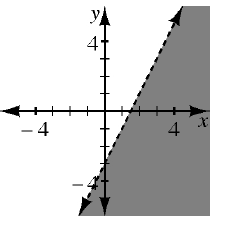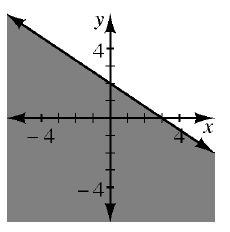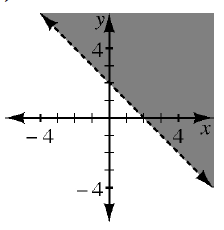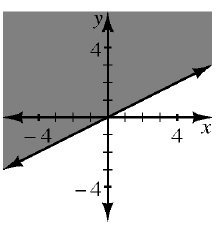### Home > CC4 > Chapter 10 > Lesson 10.2.1 > Problem10-82

10-82.

Match each graph below with the correct inequality. Homework Help ✎

1. $y>-x+2$

1. $y<2x-3$

1. $y\ge\frac{1}{2}x$

1. $y\le-\frac{2}{3}x+2$

1)2)3)4)Which graph represents
the line $y=−x+2$?

Inequality (a) matches
graph (3).

Inequality (b) matches graph (1).